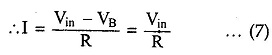# Basic Log Amplifier Using Diode

## Basic Log Amplifier Using Diode

Basic Log Amplifier Using Diode circuit diagram of basic log amplifier using a diode is shown in the Fig. 2.69.The diode D is used in the negative feedback path. The node A is grounded hence node B is at virtual ground. Hence VB = 0.As the op-amp input current is zero,Now If is the current through diode and voltage across diode isHence using equation (10) we can write,As I R is constant d.c.voltage, let us denote it as VrefWhere             Vref = Io R

Thus the output voltage V° is a function of logarithm of the input voltage Vm The circuit gives the logarithm to base e i.e., natural logarithm. But the same circuit can be used to find out logarithm values to base 10 by the proper scaling as,